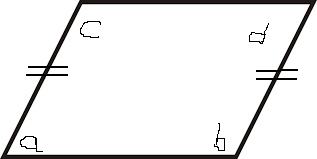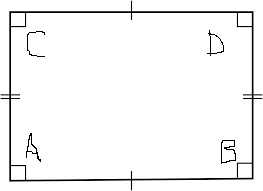## Rectangle Calculator

Calculate the various properties of rectangle or parallelogram for given values.
 Area of Parallelogramor Rectangle:[ base×height ] Enter the lengths = Area of Rectangle =

 Perimeter of Parallelogramor Rectangle:[ 2(base) + 2(height) ] Enter the lengths = Perimeter of Rectangle =

Parallelogram
In the case of parallelogram none of interior angles can not be right-angles(90°) and opposite sides are parallel and equal in length.
Rectangle
In the case of rectangle all interior angles are right angles (90°) and opposite sides are parallel and equal in length. The rectangle is also known as quadrilaterals. The diagonals of rectangle are congruent.